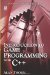# 2.6 Inequalities

## 2.6 Inequalities

Inequalities naturally extend from equations and are, as will be demonstrated later in this book, especially important for programming. Inequalities describe the relationship between numbers; not in the same way or as accurately as ratio, but more generally in terms of range. Using inequalities we can say that some number is greater than or less than another number, or that it falls anywhere in a range between two numbers, one greater and one smaller.

Inequalities use the following symbols:

• > Greater than

• < Less than

• Greater than or equal to

• Less than or equal to

### 2.6.1 Expressing Inequalities

The equation 3<9 means "3 is less than 9," and 7>1 means "7 is greater than 1." The arrows between the numbers can be thought to point in a direction along the number line; that is, greater numbers are farther to the right (>) and lower numbers are farther to the left (<). Inequalities can also be expressed with variables such as x < 7, meaning "x is less than 7"; in this case we do not know what number x is. It could be 0, 5, or even 9. All it states is that x, whatever it is, is less than 7.

In a similar fashion, the greater than or equal to and less than or equal to symbols can also be used. x 9 means x is less than or equal to 9. x could be less than 9, or it could be equal to 9, but it cannot be more than 9. The reverse is true for the greater than or equal to symbol.

### 2.6.2 Compound Inequalities

When two inequalities are combined, the result is called a compound inequality. For example, x > 3 or x < 9 means "x is greater than 3 OR x is less than 9." Another would be x > 3 and x < 9, meaning "x is greater than 3 AND x is less than 9." This latter example is expressing a range of values between a smaller and larger number. The variable x is between 3 and 9 but does not include those numbers. If we wanted to include any one of those numbers, we should use the greater than or equal to ()/less than or equal to () symbols.

### 2.6.3 Chained/Continued Inequalities

The combined inequality of AND is so prevalent it has its own notation. This is called a chained or continued inequality. Consider the following inequality:

• 3 < x < 9

Each term has a relation to the one on the right. 3 is less than x AND x is less than 9. In short, this means x is greater than 3 and x is less than 9.

### 2.6.4 Solving Inequalities

Inequalities can be expressed and solved in a similar fashion to linear equations. Such inequalities are known as linear equalities.

• 2x + 20 < 30

• 2x < 30 20

• 2x < 10

• x < 5

This inequality was solved in the same way as an equation. In the case above, x is some number less than 5. Let's consider some facts about inequalities.

• Adding or subtracting the same number on both sides

If the same number is added to or subtracted from to both sides of the inequality, the sign of the inequality will not change. For example:

• A > B

• A + 5 > B + 5

Or:

• A > B

• A 5 > B 5

• Multiplying both sides by a positive number

If both sides are multiplied by the same positive number, then the sign of the inequality will not change. The same applies for division since division can be considered as multiplication by the reciprocal.

• A > B

• A × 2 > B × 2

Or:

• A > B

• A × ½ > B × ½

• Multiplying by a negative number

If both sides are multiplied by the same negative number, then the sign of the inequality will change; otherwise the inequality will be incorrect.

• A > B

• A × 5 < B × 5

To illustrate further, let's try an example with some values.

• 5 > 4

• 5 × 5 < 4 × 5

Because:

• 5 × 5 = 25

• 4 × 5 = 20

• 25 < 20 (25 is farther left on the number line than 20)

• Inverting signs

If both sides have their signs inverted, from positive to negative or the reverse, then the sign of the inequality changes.

• A > B

• A < BIntroduction to Game Programming with C++ (Wordware Game Developers Library)
ISBN: 1598220322
EAN: 2147483647
Year: 2007
Pages: 225
Authors: Alan Thorn

Similar book on Amazon